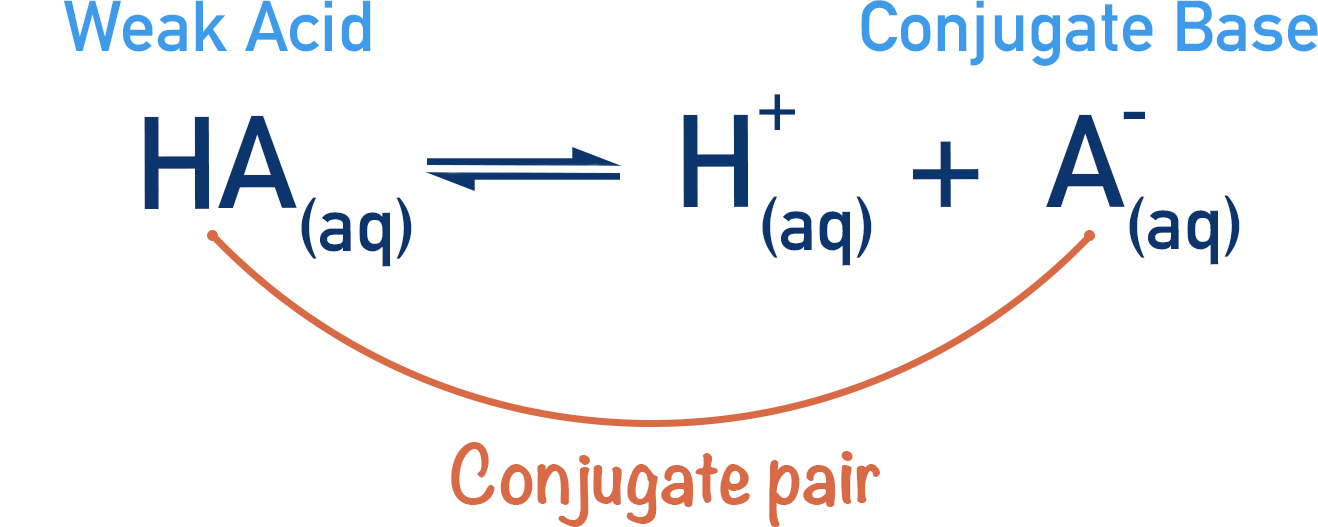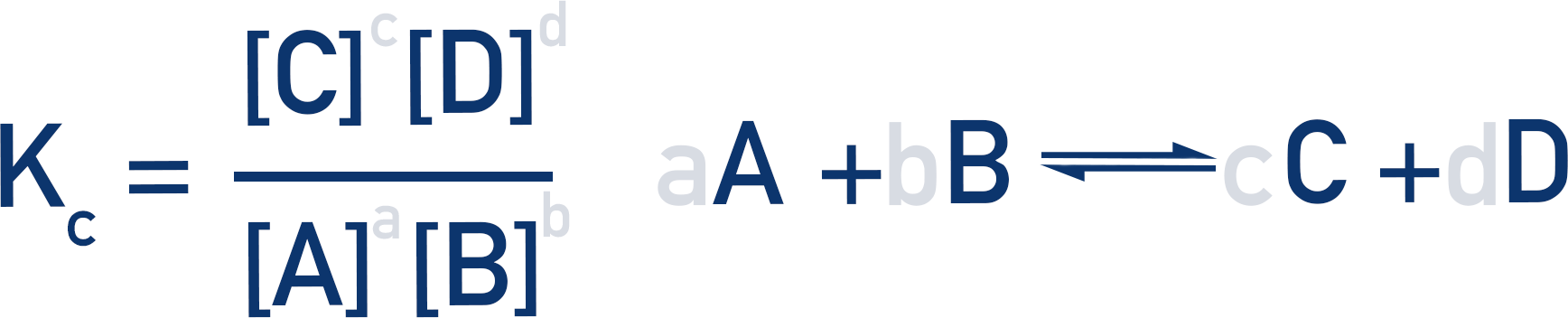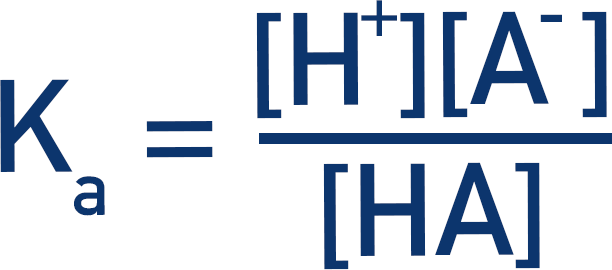Video Tutorial Electron Orbitals

Quick Notes Acid Dissociation Constant, Ka

• Weak acids only partially dissociate in solution, forming an equilibrium between the acid and its conjugate base.• Acid dissociation constants, Ka, are used to show how much either the forward or reverse reaction is favoured and to give an indication of the acid’s strength.
• If a weak acid is shown as HA and its conjugate base as A-, then the Ka for that acid can be found using:• The greater the value of Ka, the stronger the acid.
• The smaller the value of Ka, the weaker the acid.

Full Notes Acid Dissociation Constant, Ka

When acids are placed in solution they dissociate (split apart) and release H+(aq) ions. Weak acids partially dissociate, so there is an equilibrium established between the acid, its conjugate base and the released H+(aq) ions in solution.The stronger the acid, the more the position of equilibrium will favour the forward reaction (dissociation of the acid), so the concentration of H+(aq) ions in the solution will be higher.

The greater the concentration of products compared to reactants, the further the position of equilibrium lies in the forward direction. See Equilibrium.

Equilibrium constants (Kc) can be used to show where the position of equilibrium lies for a reversible reaction.We can use such an expression for the dissociation of an acid.

The acid here will be represented as HA(aq). Instead of referring to Kc, however, we will refer to Ka (acid dissociation constant). The theory is exactly the same as a Kc expression though.

HA is used to show the acid as it represents the two components of an acid: H+(aq) ions (the H in the HA) and its conjugate base, A- (the A in the HA).Then the Ka expression would be:The greater the concentration of H+(aq) ions, the stronger the acid and the larger the value of Ka.

Just like with Kc constants, the value of Ka is temperature dependant. If the temperature is changed, then the Ka value will also be different for a particular acid.